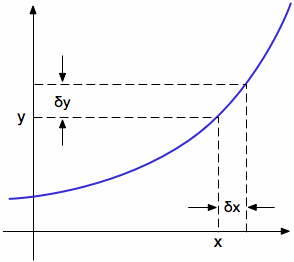•http://facebook.com/
•https://www.google.com/accounts/o8/id
•https://me.yahoo.com

# Differentiation

Differentiation of Simple Algebraic Functions from first principles

## Differentiation From First Principles

It is sometimes required that Differentiation be carried out from first principles.

Consider the following equation

Let there be small increase in x of and let the corresponding increase in y beRewriting the original equation

Multiplying out
But we know that

Subtracting equation (1) from Equation (2)

As the value is reduced and tends towards dx i.e. until it is infinitesimally small, the value of tends towards zero and can be neglected. is now written as .

It can be seen from the diagram that the value of the tangent at x,y is and at the limit this is written as

Referring to Equation (1) the value of the tangent is given by:

## The Differential Coefficient (gradient Function)

is known as the Gradient function and represents the Derivative of y with respect of x. It is also known as the b{Differential Coefficient}.

In the simplest case, if
then

Example:
##### Example - Differentiation
Problem
Find the differentiation of
Workings
Bringing the power of each x variable down, and subtracting 1 from each power of x yields:

which is simplified further to
Solution

## The Differentiation 0f A Product Of Two Functions Of X

It is obvious, that by taking two simple factors such as 5 X 8 that the total increase in the product is Not obtained by multiplying together the increases of the separate factors and therefore the Differential Coefficient is not equal to the product of the d.c's of its factors. If
then

To Prove the Product Rule let where u and v are both functions of x. Thus when x increases to u and v will also change to and .

Their product y will therefore become

Therefore , the increase in Thus

In the limit as , and tend to zero, so the above equation becomes:
Example:
##### Example - Differentiation - Product Rule
Problem
Differentiate
using the Product Rule
Workings
if
then the differential is

So for
Thus
Solution

## The Differentiation Of A Product Of Any Number Of Functions Of X

The rule for finding the differential coefficient of a product of two functions of x can be extended to apply to the product of any finite numbers of functions of x If
Where u, v, w are all functions of x, then regarding this as the product of the two factors u and w:

And similarly for any finite number of factors.

Note An important result follows from the above rules. The differential coefficient of with respect to x can be considered to be the product of two factors each of x and hence is given by:

Similarly, if n is any interger, by taking the product of n factors each of y
The differential coefficient of With respect to

## The Differentiation Of A Quotient Of Two Functions Of X

Let
Then

The proof from first principles of the Quotient Rule.

As with previous proofs from first principles x becomes , u becomes and v becomes Therefore y becomes Thus
Therefore

In the limit when tends to zero the so will and Then

Example:
##### Example - Simple example
Problem
Differentiate using the quotient rule
Workings
Then
Solution

Last Modified: 25 Jan 11 @ 19:39     Page Rendered: 2022-03-14 15:52:03